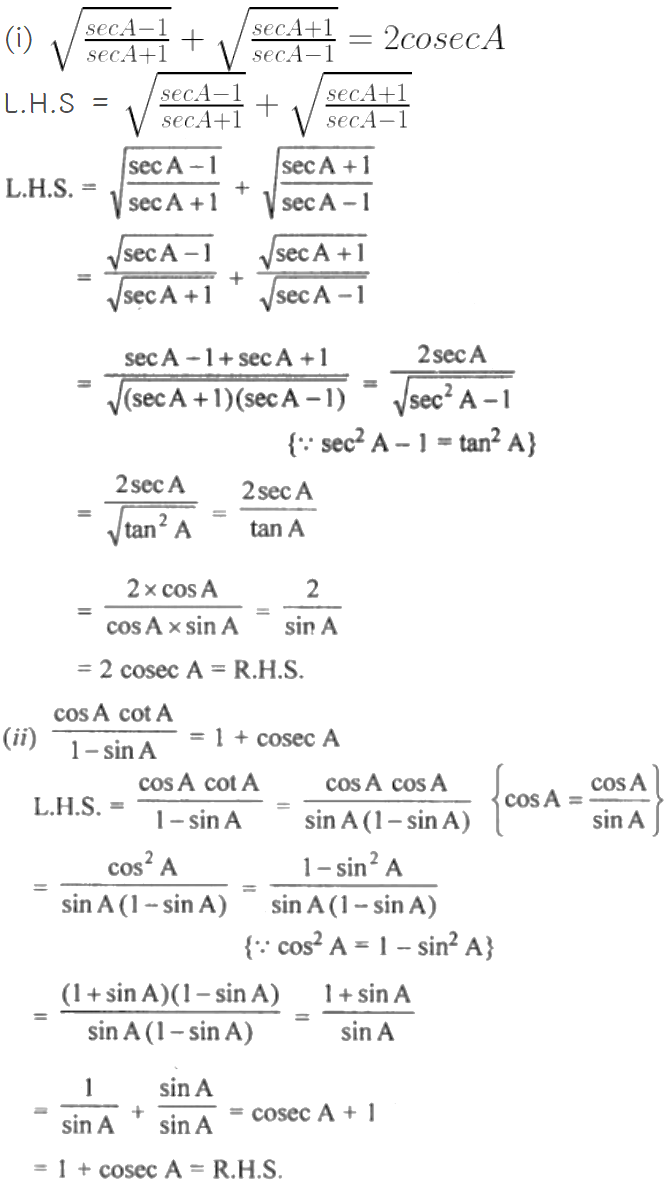Guru

# (i) Root{(secA-1) /(secA+1)} + Root{(secA+1) /(secA-1) = 2cosecA (ii) cos A cot A/ (1 – sin A) = 1 + cosec A

• 0

This is an important question from the
Book- ML Aggarwal
Board- ICSE
Publication- Avichal
Chapter- Trigonometric Identities
Chapter number-18

We have to prove that

(i) Root{(secA-1) /(secA+1)} + Root{(secA+1) /(secA-1) = 2cosecA (ii) cos A cot A/ (1 – sin A) = 1 + cosec A

Trigonometric Identities, class 10th, chapter 18th, question no 24 , ICSE board, ML aggarwal..

Share